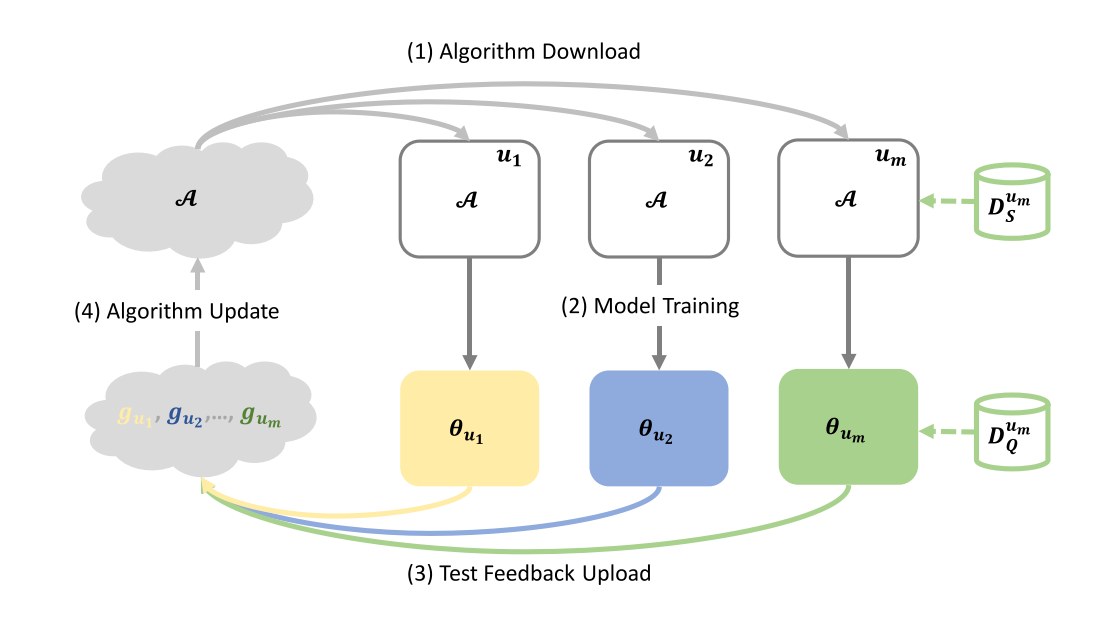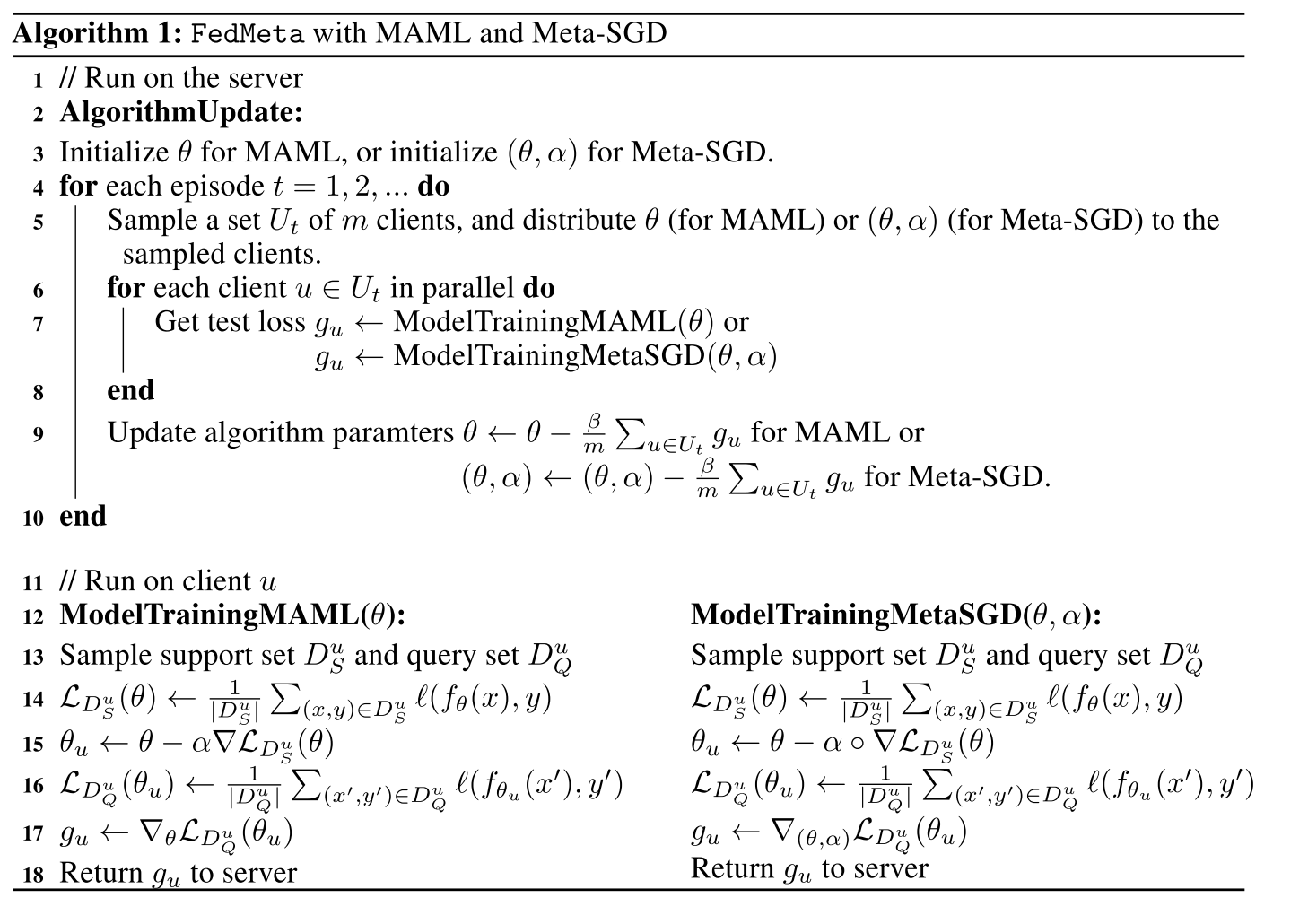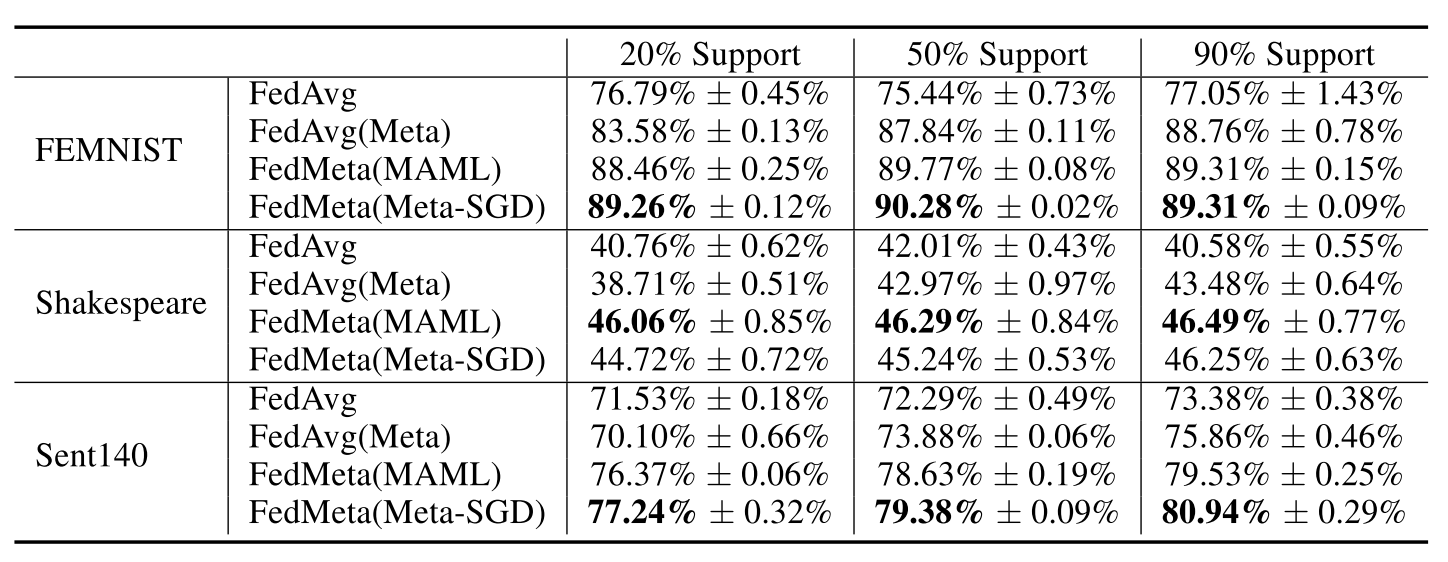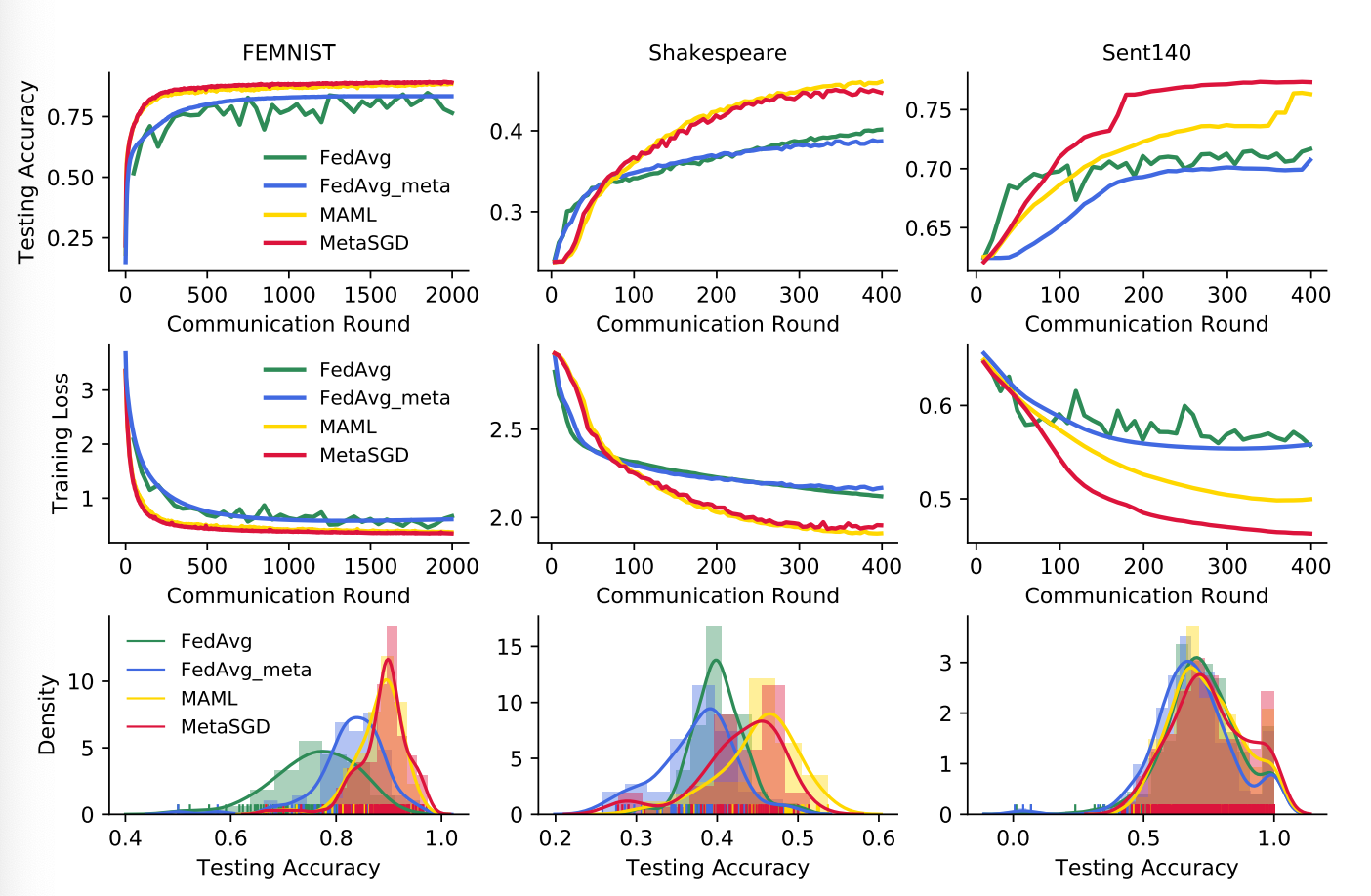• English：

# Federated Meta-Learning with Fast Convergence and Efficient Communication

## Introduction

task通常由support set和query set组成，彼此不想交。在support set上训练特定任务的模型，在query set上进行测试。然后利用测试结果更新算法。在联邦元学习中，算法（meta learner）在server端维护，并分发到clients进行模型的训练。元训练的每个episode过程中，被采样到的一个batch的clients会接受到算法（meta learner）的参数然后进行模型训练。然后将query set上的测试结果上传到server端更新 meta learner。整体流程如图所示：20200218221422.png

Comparing federated meta-learning with federated learning FML和ML的比较。 联邦元学习类似于联邦学习，区别主要在于共享的不再是全局模型，而是算法参数（元模型参数）。但是，元学习在概念上和分布式模型训练不同，而且在共享元学习算法可以比共享模型更灵活应用。例如在图像分类中，n个类别的图像可能在clients之间不均匀地分布，其中每个client最多具有k个类别。对于联邦学习需要训练一个大型的n类分类器，以利用来自所有客户端的数据，然而其实k类分类器就足够了，因为他每次其实只为一个client做预测。对于联邦学习这种庞大的模型需要大量的通信成本，虽然可以只向client发送模型的一部分以更新相关参数，但这必须先了解client的私有数据才能决定。而另一方面，在元学习中，算法可以训练包含不同类别的任务。例如MAML可以通过对k类任务进行元训练来为K类分类器提供初始化，无论具体类别是什么。因此，在FML框架中，可以利用MAML对所有n个类别进行k类分类器初始化的元训练。也就是让一个k类分类器在n个类别的任务背景下进行初始化的元学习。这样FML的通信和计算成本都降低了。

Contributions 本文贡献主要专注于联邦学习设置方面的算法设计，为此本文提出了一个新的框架并做了大量的实验。贡献点主要有三：1. 提出FedMeta框架，结合meta和fed，将maml算法和meta-sgd集成到federated learnin中。2. 在LEAF数据集上进行试验，与FedAvg进行比较，结果表明Fedmeta减少开销，同时精度更高。3. 将FedMeta应用于推荐任务，其中每个client都有高度personalized的记录，通过实验证明，与独立或联邦学习方法系那个比，元学习算法可以实现更高准确性。

## Federated Meta-Learning

### The Meta-Learning Approach

$\min _{\varphi} \mathbb{E}_{T \sim \mathcal{T}}\left[\mathcal{L}_{D_{Q}^{T}}\left(\theta_{T}\right)\right]=\min _{\varphi} \mathbb{E}_{T \sim \mathcal{T}}\left[\mathcal{L}_{D_{Q}^{T}}\left(\mathcal{A}_{\varphi}\left(D_{S}^{T}\right)\right)\right]$

MAML是具有代表性的元学习算法。对于MAML的算法A就是用于产生模型的初始状态。具体地说，就是对于每个task T，算法使得$\alpha = \theta$，使得算法的参数和模型f的参数相等。然后模型f的参数在support set上训练，根据损失函数进行更新：$\mathcal{L}_{D_{S}^{T}}(\theta):=\frac{1}{\left|D_{S}^{T}\right|} \sum_{(x, y) \in D_{S}^{T}} \ell\left(f_{\theta}(x), y\right)$。最后把模型参数在query set上进行测试，然后计算测试的损失：$\mathcal{L}_{D_{Q}^{T}}\left(\theta_{T}\right):=\frac{1}{\left|D_{Q}^{T}\right|} \sum_{\left(x^{\prime}, y^{\prime}\right) \in D_{Q}^{T}} \ell\left(f_{\theta_{T}}\left(x^{\prime}\right), y^{\prime}\right)$

$\min _{\theta} \mathbb{E}_{T \sim \mathcal{T}}\left[\mathcal{L}_{D_{Q}^{T}}\left(\theta-\alpha \nabla \mathcal{L}_{D_{S}^{T}}(\theta)\right)\right]$

$\min _{\theta, \alpha} \mathbb{E}_{T \sim \mathcal{T}}\left[\mathcal{L}_{D_{Q}^{T}}\left(\theta-\alpha \circ \nabla \mathcal{L}_{D_{S}^{T}}(\theta)\right)\right]$

### The Federated Meta-Learning Framework

FML的 目标是能够协作式的通过分布在各个clients的数据meta train一个算法。以MAML为例，就是希望那个利用所有clients的数据，来完成一个模型的初始化。MAML包括两个层次的优化过程：内循环利用初始化参数训练特定任务模型，外循环根据测试损失更新初始化参数。在联邦学习的环境中，每个client u会从服务器接收初始化参数 $\theta$，然后根据设备上的support set数据进行模型的训练，并传输test loss到server。server主要维护初始化参数，并根据clients的test loss对其进行更新。20200219111333.png

## Experiments

1. 在LEAF数据集上实验，证明了其收敛性块，准确性高。
2. 在实际环境下的推荐任务中进行实验，可以保证在较小的规模依然保持较强的适应力。

### Evaluation Scheme20200219114337.png

### LEAF Datasets

Accuracy and Convergence Comparsion 考虑到边际设备的运算能力的限制，每个client的local epoch设置为1。20200219125820.png

Fairness Comparison 本文同时还比较了多次实验，利用平均最终的准确度分布，来研究FedAvg和FedMeta。从前面的图的最后一行，展示了不同方法的核密度估计。对于FEMNIST，MAML和Meta-SGD不仅导致较高的均值，而且方差更低。对于Shakespeare数据集，FedMeta方差虽然更大，但是均值也更大。对于Sent140，准确度的分布都差不多。然而可以看出，MAML和Meta-SGD有更多接近100%的clients。总之，对于图像分类任务，FedMeta的准确度分布更为稳定集中。

## Conclusion

FedMeta框架，在准确性 收敛速度和通信成本都比原本的FedAvg要更好。